# $\xi$ and $Z$ functions

The functional equation can be written in a form which is more symmetric: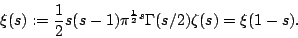Here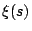is known as the Riemann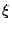-function. It is an entire function of order 1, and all of its zeros lie in the critical strip.

The-function associated to a general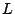-function is similar, except that the factor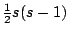is omitted, since its only purpose was to cancel the pole at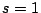.

The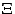function just involves a change of variables: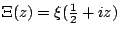. The functional equation now asserts that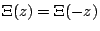.

The Hardy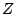-function is defined as follows. Let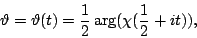and define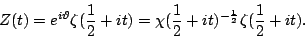Then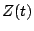is real for real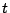, and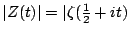.

Plots ofare a nice way to picture the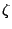-function on the critical line.is called RiemannSiegelZ[t] in Mathematica.

Back to the main index for The Riemann Hypothesis.